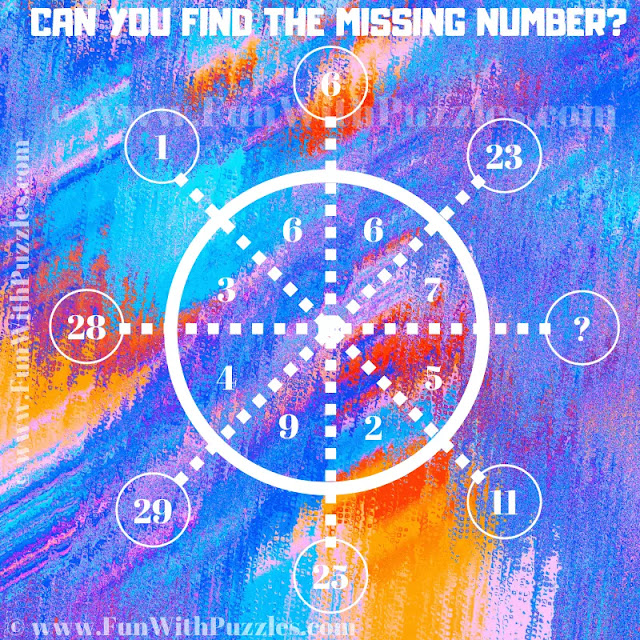This is a tough maths logical reasoning puzzle that will twist your brain. Solving this puzzle will test your mathematical skills and logical reasoning ability. In this logical puzzle, you are shown some number inside and around the Circle. These numbers follow some mathematical pattern. Your challenge in this maths logic puzzle is to find this pattern. Once you are able to crack the code, find the value of the missing number which will replace the question mark.
This is a tough reasoning puzzle. Write down your answer along with the logical reasoning in the comments box.Can you Find the Missing Number?

The answer to this "Logical Reasoning Puzzle", can be viewed by clicking on the answer button.